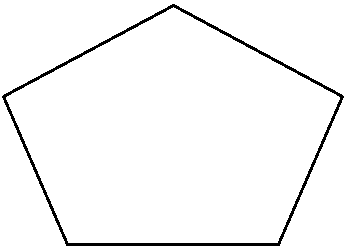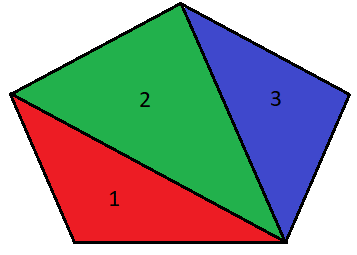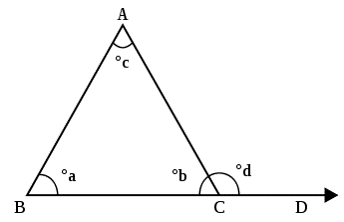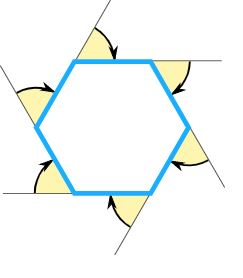# Interior/Exterior Angles

We have learnt about the interior angles inside a triangle (do you remember they add to 180?). In this lesson we will expand that knowledge to different shapes (called polygons).

## Interior Sum of Angles

The interior angles of any specific polygon always add up to a constant value, which depends only on the number of sides. So in other words, regardless of how irregular the shape is, the sum of its internal angle would remain the same.For example a pentagon has 5 sides, and its interior angles always add up to 540°, and that's simply because its shape can be made from three triangles.

$$3 × 180° = 540°$$Let's take a look at the interior angles of different shapes:

Interior angle of polygons
Shape Sides (n) Sum of Interior Angles Equiangular Angle
Triangle 3 180° 60°
Pentagon 5 540° 108°
Hexagon 6 720° 120°
Heptagon 7 900° 128.57°
Octagon 8 1080° 135°

Notice that when the side increase by one, the sum of interior angles increases by 180 (this is a linear relation!). The general rules is:

Sum of Interior Angles$$\ = (n−2) × 180°$$

If the shape is regular and each angle is the same (equiangular polygon), all angles are:

$$\ \theta = (n−2) × \cfrac{180°}{n}$$

## Exterior Sum of Angles

If the side of a polygon is extended, the angle formed outside the polygon is the exterior angle. In the figure below, angle d would be one of the exterior angle of the triangle.

Also since we are extending a side of the polygon, that exterior angle must be supplementary to the polygon's interior angle. Together, the adjacent interior and exterior angles will add to 180° (b+d=180).The general rules for exterior angle is:

Exterior Angle$$\ = 180° -$$ Interior Angle

The Exterior Angles of a polygon always add up to 360°:

Sum of Exterior Angles$$\ =$$ Exterior Angle × $$n = 360$$

This might seem strange, but think about the shape that is made if you start from one external angle and move on to the next until the end .... it makes a circle! And a circle is 360°.Given that each exterior angles are the same for each vertex on the polygon (equiangular polygon), the exterior angle can be calculated using:

$$\phi=\cfrac{360}{n}$$

A quadrilateral has interior angles of 98°, 120° and 108°. What is the measure of the missing interior angle?

Each exterior angle of a regular polygon each measures 72°. How many sides does the polygon have?

In a triangle, x, y and 50° are the three interior angles and exterior of y measures 92°. What is the measure of angle x?: